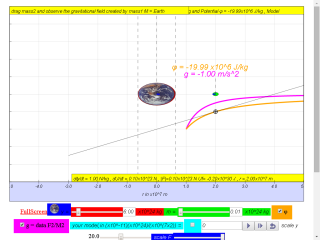gravity07_1

### 7.3.8.4.1 Values for the gravitational potential due to the Earth are given in the table below.

 Distance from Earth’s surface / m Distance from Earth’s centre / m Gravitational potential / MJ kg-1 Gravitational Field Strength g / ms-2 Rate of change of potential with distance  $\genfrac{}{}{0.1ex}{}{d\varphi }{dr}$/ ms-2 0 6 370 000 = 6.37x106= 0.637x107 -62.72 10 000 000 = 1.0x107 -40.10 1.363x107 2.0x107 -20.00 3.0x107 -13.34 -0.44 0.44 4.0x107 -10.01 5.0x107 -8.01 -0.16 0.16 Infinity 0

(i) given that radius of Earth = 6370 km, fill in the missing values in the column of Distance from Earth’s surface / m.

(ii)    Calculate the change in potential if an object travels from r = 5.0x107 m to r = 2.0x107 m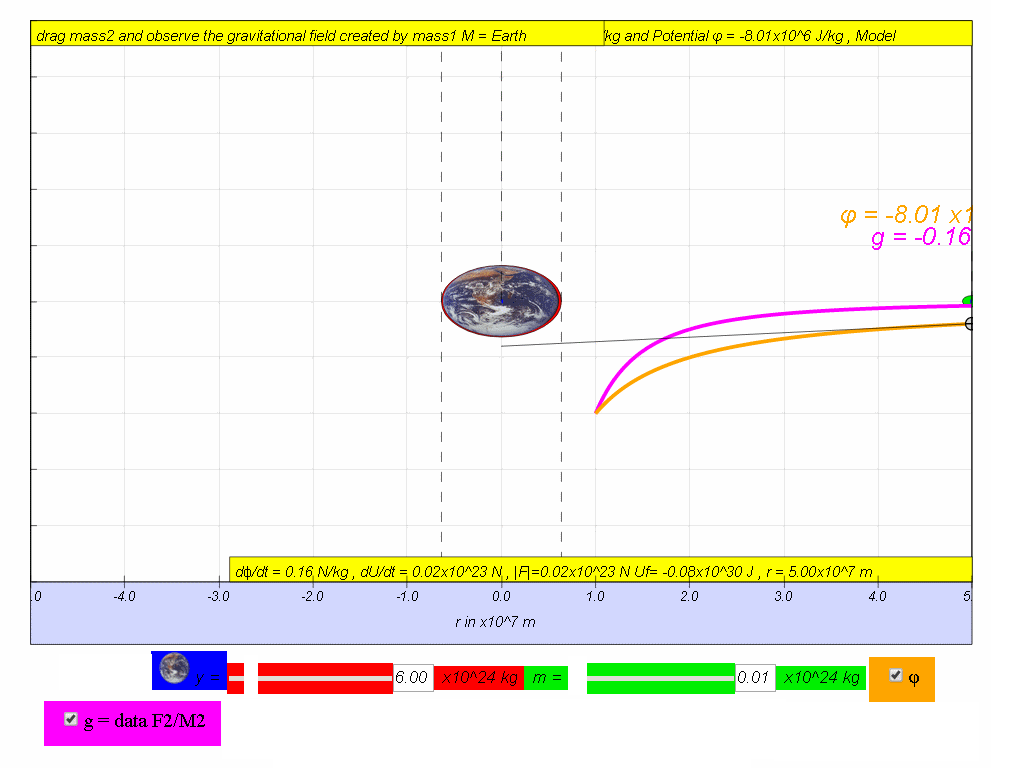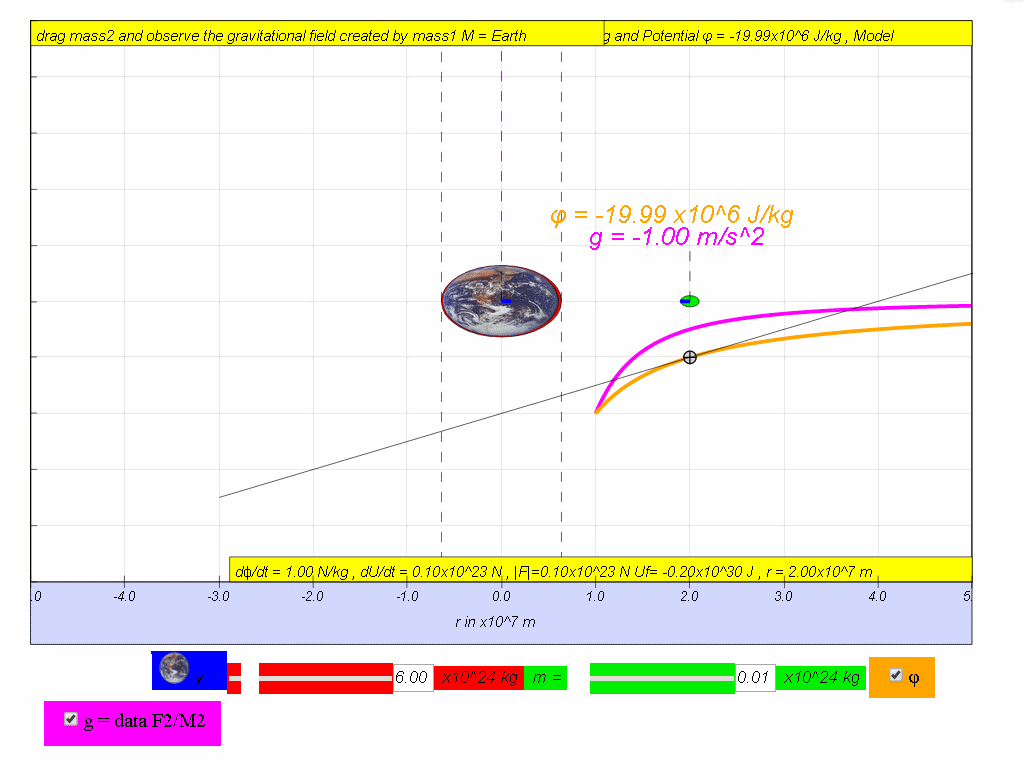Answer: Δϕ = ϕfinal - ϕinitial = -20.00x106 - (-8.01x106) = -11.95x106 J/kg

(iii) Hence or otherwise, calculate the change in potential energy if the object-satellite has a mass 0.01x1024 kg.

Answer:  ΔPE = ΔU = m(Δϕ) = 0.01x1024(-11.95x106) = -1.196x1029 J = -0.20x1030 J

(iv) Determine the potential energy lost by object-satellite of 0.07x1024 kg from a height of 13 630 000 to the Earth's surface.

Answer: ΔPE = m(Δϕ) = 0.07x1024 (-6.67x10-11)(6.0x1024)($\frac{1}{\left(6.37x1{0}^{6}\right)}-\frac{1}{\left(13630000+6.37x1{0}^{6}\right)}$ ) = (0.07x1024 )(-6.27x107 -(-20.00x107))= (0.07x1024 )(-4.28x107 ) = 2.996x1030 = 3.00x1030 J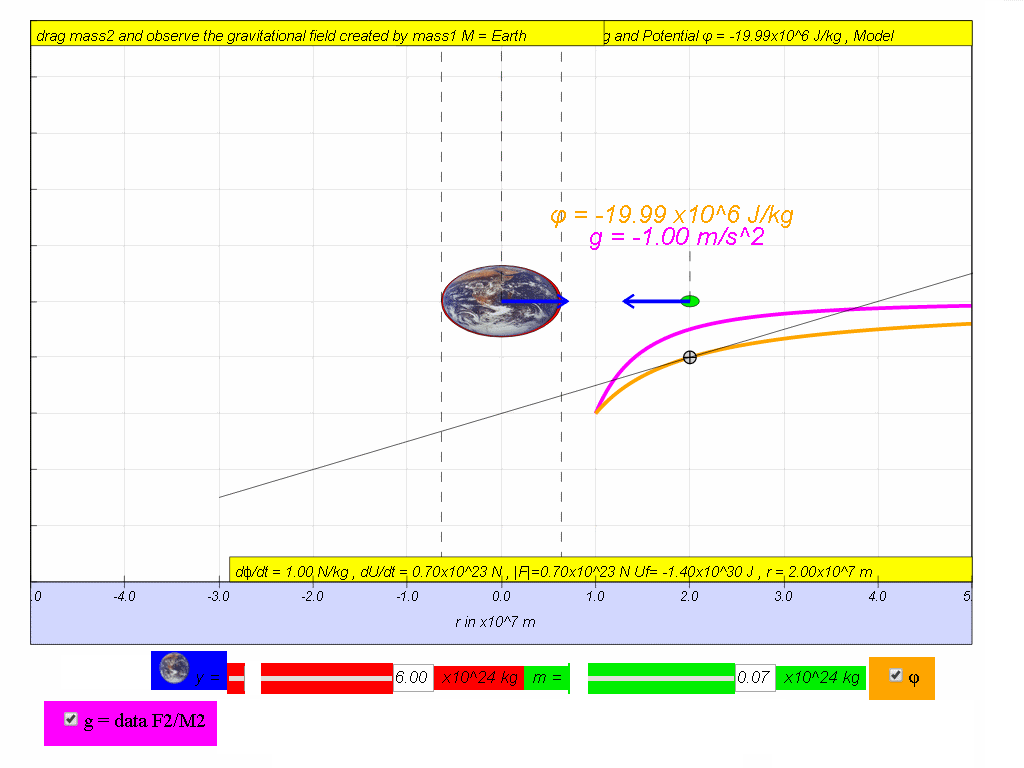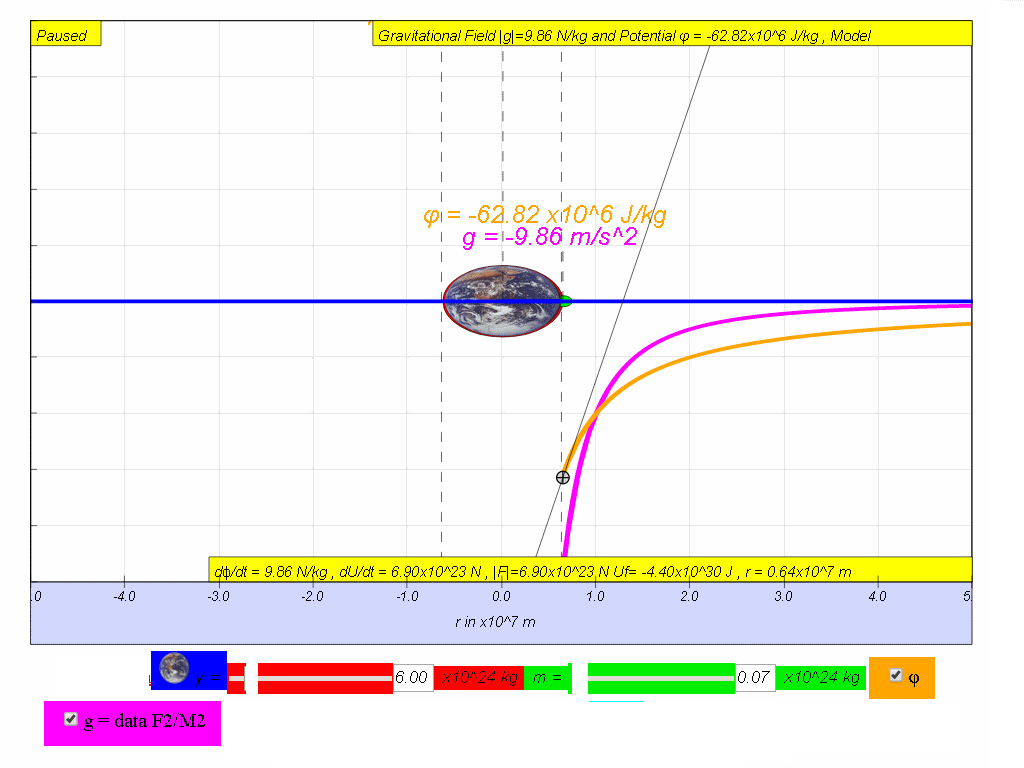v) by means of using the simulation, move the mass and record down and fill in the last 2 missing columns for Gravitational Field Strength and  rate of change of potential with distance.

vi) Hence, suggest a relationship between g, ϕ and r.

vii) Explain why the accuracy of determining g at r = 3x107 is poor when using the following values at r = 2x107 and 4x107 m where $\genfrac{}{}{0.1ex}{}{d\varphi }{dr}=-\frac{-10.01-\left(20.00\right)}{4x1{0}^{7}-2x1{0}^{7}}$

### Translations

Code Language Translator Run### Software Requirements

SoftwareRequirements

 Android iOS Windows MacOS with best with Chrome Chrome Chrome Chrome support full-screen? Yes. Chrome/Opera No. Firefox/ Samsung Internet Not yet Yes Yes cannot work on some mobile browser that don't understand JavaScript such as..... cannot work on Internet Explorer 9 and below

### CreditsThis email address is being protected from spambots. You need JavaScript enabled to view it.

### end faq

Rating 5.00 (2 Votes)